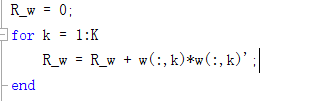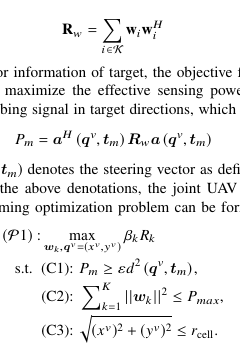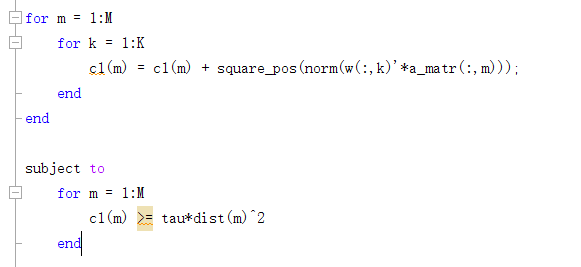# How to deal with the product of column vector and its conjugate transpose?

Thank you for your contribution to the development and application of CVX. As a newcomer, I have a question to expect your answer.
In the constraint condition of an optimization problem, I want to set R _ w > = a constant, where R _ w is defined as follows figure, and w is a complex column vector ;I program in the CVX environment in MATLAB as shown below, but CVX error : Only scalar quadratic forms can be specified in CVXHow can I deal with the product of column vector and its conjugate transpose?

CVX does not allow the formation of that product.

However, depending on what you want to do with the resulting covariance matrix, there may be a way to accomplish that without explicitly forming the covariance matrix. For instance,`x'*w*w'*x = square_pos(norm(w'*x))`, which will be accepted by CVX (presuming `w` is a CVX variable and x is input data). And if that term appears by itself in the objective function. there is no need to apply `square_pos`.

Thanks for your reply. I tried the method you said and then passed the form check from CVX.
In fact, for this covariance matrix, I hope that it can be greater than a constant in the constraint, that is, a ’ * R _ w * a > = c, in the form shown below.I used square _ pos ( norm ( w ( :, k ) ’ * a ) ) in MATLAB, as shown in the following figureHowever, CVX prompts errors：
Invalid constraint: {convex} >= {real constant}
How should I solve this problem Is this optimization problem nonconvex? The objective and constraint (C2) are checked and have no fault, the (C3) is ignored in this problem.

The constraint in your CVX code is non-convex, because the inequality is going the wrong direction to be convex. concave >= affine is convex. convex <= affine is convex. The reverse direction nequalities are non-convex.

I can’t say for sure that (c1) is non-convex because I’m not clear on what are optimization variables and what’s input data. That’s for you tro figure out.

Before trying to use CVX, you should read the CVX User’s Guide. Perhaps you should also study the first several chapters of https://web.stanford.edu/~boyd/cvxbook/ .

Start by carefully reading Why isn't CVX accepting my model? READ THIS FIRST! which you either didn’t do or didn’t understand when I posted the link earlier.